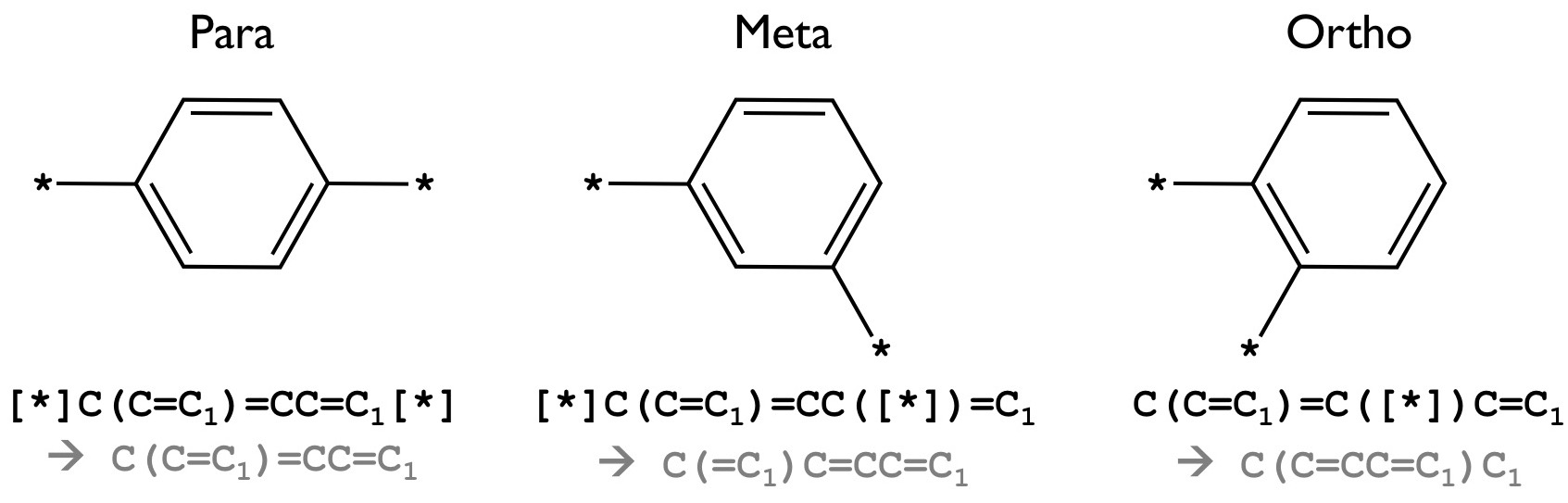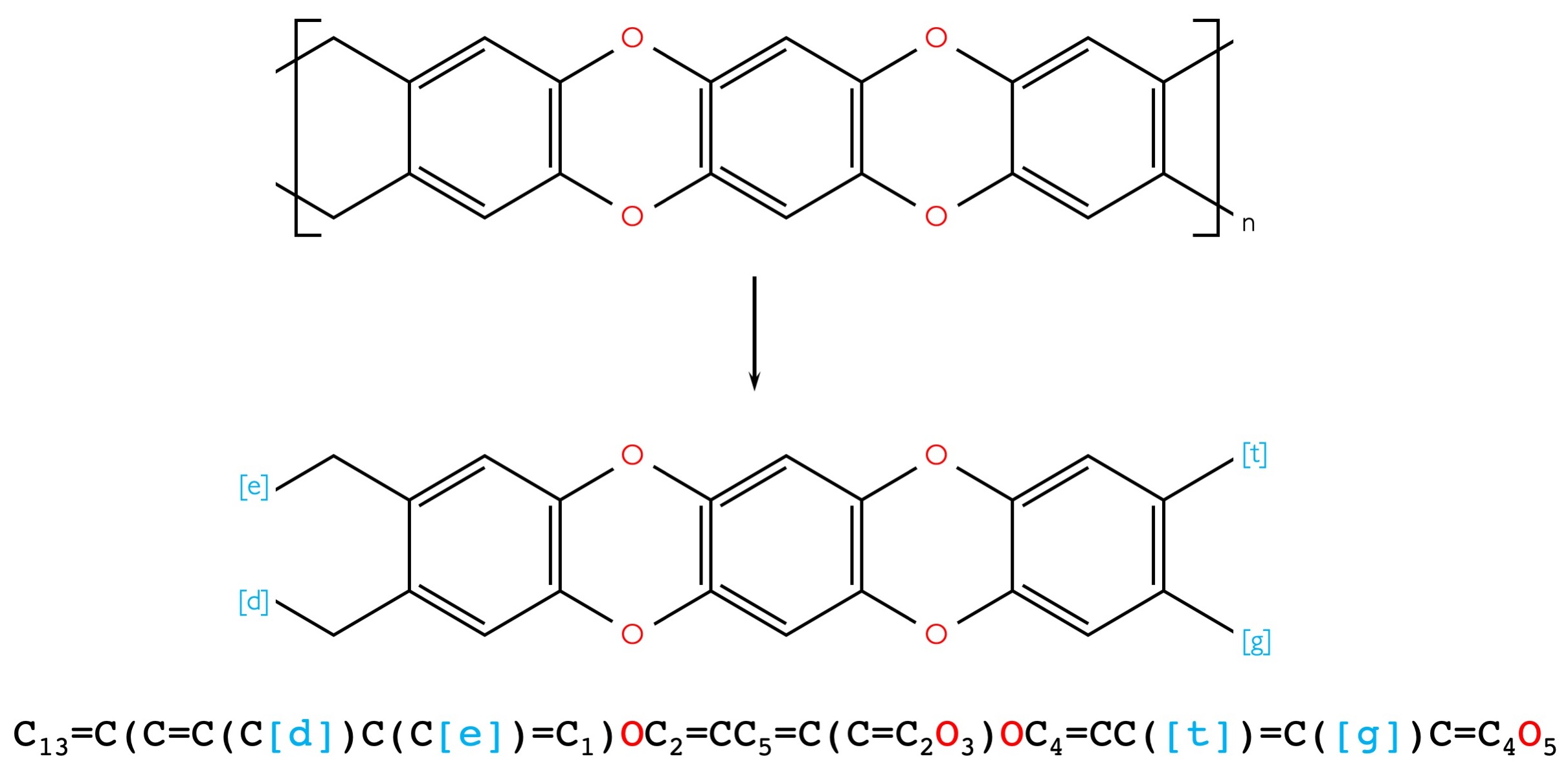### SMILES guidelines

SMILES (simplified molecular-input line-entry system) uses short ASCII string to represent the structure of chemical species. Because the SMILES format described here is custom-designed by us for polymers, it is not completely identical to other SMILES formats. Strictly following the rules explained below is crucial for having correct results. Details of the rules are given below, while the SMILES strings of some example polymer blocks and polymers are provided in Table 1.

• Spaces are not permitted in a SMILES string.
• An atom is represented by its respective atomic symbol. In case of 2-character atomic symbol, it is placed between two square brackets `[ ]`.
• Single bonds are implied by placing atoms next to each other. A double bond is represented by the = symbol while a triple bond is represented by `#`.
• Hydrogen atoms are suppressed, i.e., the polymer blocks are represented without hydrogen. Polymer Genome interface assumes typical valence of each atom type. If enough bonds are not identified by the user through SMILES notation, the dangling bonds will be automatically saturated by hydrogen atoms.
• Branches are placed between a pair of round brackets `( )`, and are assumed to attach to the atom right before the opening round bracket `(`.
• Numbers are used to identify the opening and closing of rings of atoms. For example, in `C1CCCCC1`, the first carbon having a number "1" should be connected by a single bond with the last carbon, also having a number "1". Polymer blocks that have multiple rings may be identified by using different, consecutive numbers for each ring.
• Atoms in aromatic rings can be specified by lower case letters. As an example, benzene ring can be written as `c1ccccc1` which is equivalent to `C(C=C1)=CC=C1`.
• A SMILES string used for Polymer Genome represents the repeating unit of a polymer, which has 2 dangling bonds for linking with the next repeating units. It is assumed that the repeating unit starts from the first atom of the SMILES string and ends at the last atom of the string. These two bonds must be the same due to the periodicity. It can be single, double, or triple, and the type of this bond must be indicated for the first atom. For the last atom, this is not needed. As an example, `CC` represents -CH2-CH2- while `=CC` represents `=CH-CH=`.
• Atoms other than the first and last can also be assigned as the linking atoms by adding special symbol, `[*]`. As an example, `C(C=C1)=CC=C1` represents poly(p-phenylene) with link through para positions, while `[*]C(C=C1)=CC([*])=C1` and `C(C=C1)=C([*])C=C1` have connecting positions at meta and ortho positions, respectively (see Figure 1).Figure 1: Example of SMILES using [*] to specify a connection point. Equivalent SMILES without [*] is shown together.

Table 1: SMILES strings of the polymer blocks considered and some polymers constructed from them.
 Chemical formular SMILES -CH2- `C` -NH- `N` -CS- `C(=S)` -CO- `C(=O)` -CF2- `C(F)(F)` -O- `O` -C6H4- `C(C=C1)=CC=C1` -C4H2S- `C1=CSC(=C1)` -C5H3N- `C1=NC=C(C=C1)` -C4H3N- `C(N1)=CC=C1` -CH2-NH-CO-CH2- `CNC(=O)C` -CH2-C6H4-C4H2S-C6H4- `CC(C=C1)=CC=C1C2=CSC(=C2)C(C=C3)=CC=C3` -NH-CO-NH-C6H4- ` NC(=O)NC(C=C1)=CC=C1` -CO-NH-CO-C6H4- `C(=O)NC(=O)C(C=C1)=CC=C1` -NH-CS-NH-C6H4- `NC(=S)NC(C=C1)=CC=C1`

A ladder polymer is a type of double stranded polymer with multiple connection points between monomer repeat units. Different from typical polymers the ladder polymer requires four different symbols (`[e]`, `[d]`, `[t]` and `[g]`) to specify the connection points between monomers. A point `[e]` is assumed to be connected to a point `[t]` of the next monomer. (and `[d]` connected to `[g]`) Figure 2 shows an example ladder polymer and how it could be input using Polymer Genome Drawing tool.